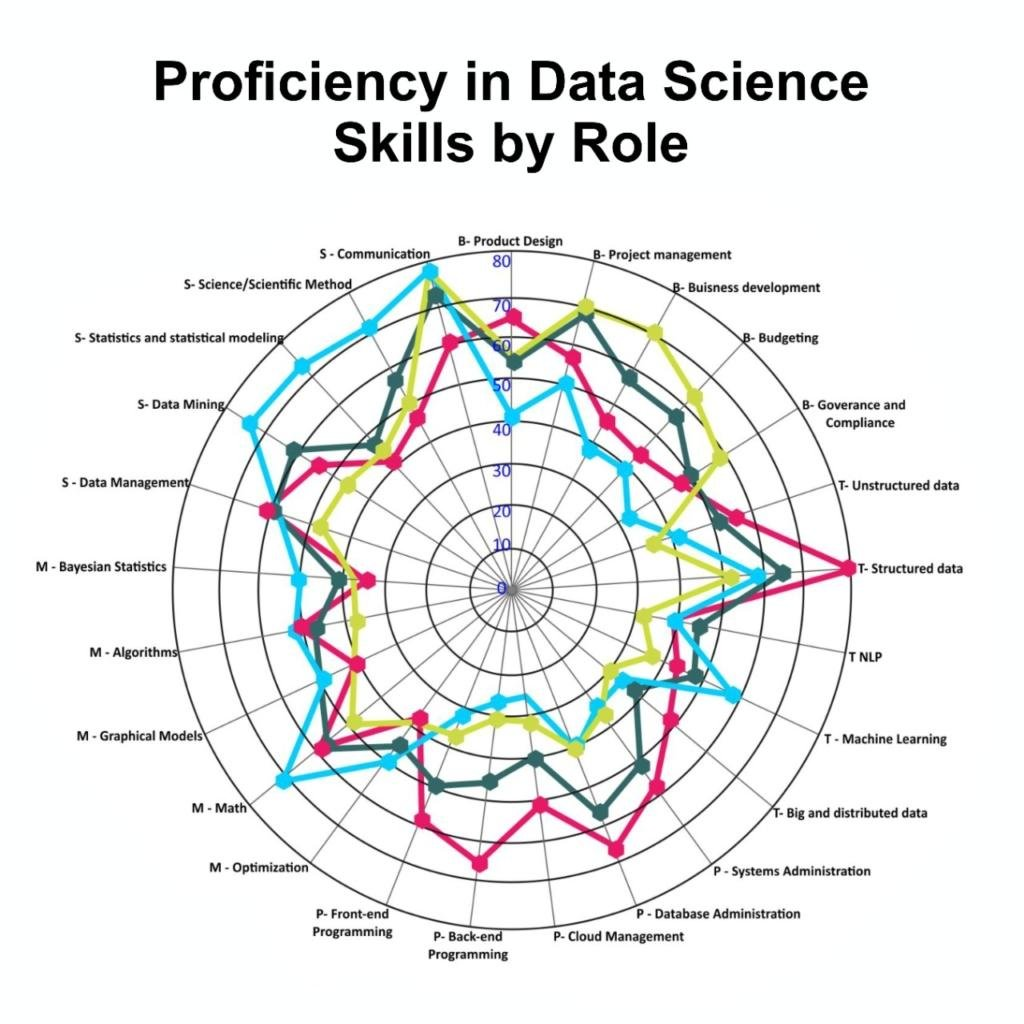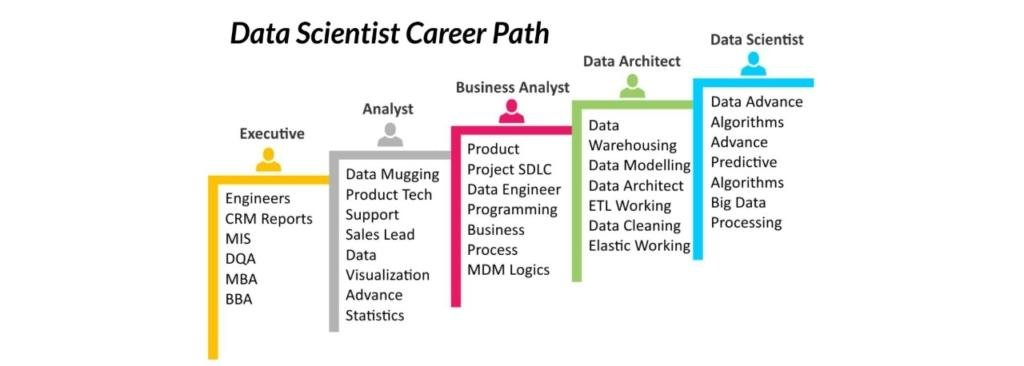# Description

Data science is a field of study that involves the analysis and processing of large amounts of data to uncover patterns, trends, and insights. It is the process of transforming raw data into meaningful information and insights, which can be used for decision making. Data science is a multidisciplinary field that incorporates techniques from computer science, mathematics, and statistics, which are used to discover patterns and uncover insights in data. Data science is used to identify relationships between variables and to predict future outcomes.

Data science is a rapidly growing field that applies the principles of mathematics, statistics, computer science, and machine learning to the analysis of structured and unstructured data. Data science is used to uncover insights from data, such as customer preferences, trends in customer behavior, or customer sentiment. It is also used to build predictive models to forecast future outcomes or to make decisions.# Course Syllabus

This program’s curriculum is comprehensively designed to help learners acquire data science competencies while working with hands-on projects using prominent technologies like Python, MongoDB, Pandas, Numpy, Tableau & PowerBi Data Methods. Join the league of Emerging Data Scientists who utilize their best skills to find the best trends and to manage the data as analytical Data Experts.

1. Introduction to Jupyter Notebook
2. Getting Started with Data Science
3. Unix Introduction
1. Python Basics
2. Python Introduction
3. Python Data Structure: Lists and Arrays
4. Python : Conditions and Branching
5. Python : Functions and Methods
6. Python : Objects and Classes
7. Practice Questions in Python
8. Introduction to NumPy
9. Linear Algebra in NumPy
10. Seaborn, Matplotlib
11. Project 1 :Satellite Image Data Analysis using NumPy
12. Introduction to Pandas
1. Introduction to Probability Theory
2. Operation in Sets
3. Permutations and Combinations
4. Probability : Programming in Python
5. Conditional Probability
6. Continuous Probability Distributions (Gaussian, Exponential)
7. Central Limit Theorem (Introduction)
8. Estimating the Sample Mean
9. 2D data Plots and Analysis
10. Plotting Probability Distributions (Histogram, BoxPlot)
11. T Test/Z Test/Anova
1. Data Acquisition
2. Data Wrangling
3. Data Statistical Analysis, Grouping and Correlation
4. Model Development
5. Model Evaluation and Refinement
6. Getting started in scikit-learn with the famous iris dataset
7. Training a Machine Learning Model with scikit-learn
8. Comparing Machine Learning Models in scikit-learn
9. Data Science Pipeline: Pandas, Seaborn, and scikit-learn
10. Cross-Validation for Parameter Tuning, Model Selection, and Feature Selection
11. Efficiently Searching for Optimal Tuning Parameters
12. Evaluating a Classification Model: Confusion Matrix and ROC

1. Data Acquisition
2. Data Wrangling
3. Data Statistical Analysis, Grouping and Correlation
4. Model Development
5. Model Evaluation and Refinement
6. Getting started in scikit-learn with the famous iris dataset
7. Training a Machine Learning Model with scikit-learn
8. Comparing Machine Learning Models in scikit-learn
9. Data Science Pipeline: Pandas, Seaborn, and scikit-learn
10. Cross-Validation for Parameter Tuning, Model Selection, and Feature Selection
11. Efficiently Searching for Optimal Tuning Parameters
12. Evaluating a Classification Model: Confusion Matrix and ROC

1. Stemming, Phrase identification, word sense disambiguate
2. POS tagging
3. TF and IDF
4. N-gram models of language
5. Word to Vector, Doc to Vector
6. Applying ML Algorithms
1. Simple Linear Regression
2. Multiple Linear Regression
3. Non-Linear Regression
4. Regression Methods
5. Ridge Regression and Lasso Regression
6. Linear Regression and Decision Tree Regression
7. Random Forest Regression
8. Logistic Regression
9. Project 4 : Sentiment Analysis using Logistic Regression
10. Decision Tree Classification
11. Project 5 : Daily Weather Data Analysis using Decision Tree Classification
12. Random Forest Classification
13. Boosting Algorithms
14. Bagging
15. K- Nearest Neighbours Classification
16. Project 6 : Nearest Neighbour for Handwritten Digit Recognition

17. ethical Clustering on the same dataset

18. Hierarchical Clustering

19. Project 7 : Minute Weather Data Clustering using K-Means Clusterin

20. Density-Based Spatial Clustering of Applications with Noise (DB-SCAN)

21. K-Means and Hierarc

22. g

23. K-Means Clustering

24. Naive Bayes Classification

1. Evaluate the speed, runtime and memory dependencies of algorithmic models
2. Parallel computing systems such as SISD (Single Instruction SingleData Stream), SIMD (Single Instruction Multiple Data Streams),MISD (Multiplelnstructions Single Data Stream), MIMD (Multiple Instructions Multiple DataStreams)
3. How to use coding tools
4. Create, review and execute unit test cases
5. Corrective and Preventive actions for problems and defects can improve future designs
6. Measure and Optimize performance of algorithm
7. Deployment of the Models
1. RDBMS Principals
2. Install a DB Engine
3. SQL syntax and Data types Operators, Expressions, Comments
4. Data Definition Language (DDL) Data Manipulation Language (DML)
5. Grant and Revoke
6. SQL Functions (Sum , Count, Avg etc)
7. Joins (self, left, right, full outer)
8. Queries and Sub Queries
9. SQL Clauses
10. SQL Window functions
11. SQL Real time examples
12. SQLlive Practice Session
1. Tableau Desktop
2. Tableau Products
3. Tableau Terminology
4. Data Connection
5. Working with Data
6. Visualizing Data
7. Statistical Models
8. Dashboards
9. Sharing the Visuals

# Data Science career opportunities include:

1. Data Analyst: Analyzing data sets to identify patterns and trends and make data-driven decisions.
2. Data Engineer: Designing, building, and maintaining data infrastructure, such as databases and data warehouses.
3. Machine Learning Engineer: Creating algorithms and models to automate data analysis and predictions.
5. Data Scientist: Combining computer science, statistics, and machine learning to solve complex problems.
6. Data Visualization Developer: Creating visual representations of data to help stakeholders understand and make decisions.
7. Data Strategist: Developing strategies around data collection, storage, and analysis.
8. Natural Language Processing Scientist: Developing algorithms to process and interpret natural language.
9. AI Developer: Building artificial intelligence systems for data science applications.
10. Big Data Engineer: Developing systems to process and analyze large amounts of data.# role of a data scientist:

The role of a data scientist is now a buzzworthy career. It has staying power in the marketplace and provides opportunities for people who study data science to make valuable contributions to their companies and societies at large. Here are six things that should make you realize data science is the career of the future.
Companies Struggle to Manage Their Data
New Data Privacy Regulations Increase the Need for Data Scientists
Data Science Is Still Evolving
Data Scientists Have In-Demand Skills
A Staggering Amount of Data Growth
High Likelihood of Career Advancement Opportunities